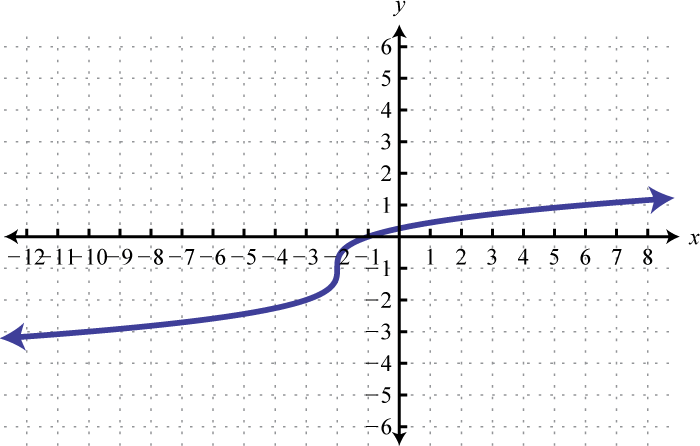Name that parent function
Describe the Translation
Equation from the Graph
Miscellaneous
Write the equation
100

this parent function's graph looks like a "V"

What is absolute value

100

f(x) +2

What is translated up 2

100What is cuberoot(x+2) -1

100

What is the average rate of change for the function f(x)= 2x+1 from the interval of -5 to 2

What is 2

100

Quadratic function moved up 7 units

What is f(x) = x^2 + 7

200

this parent function's graph is "U" shaped

200

f(x) = (x + 7)

What is translated left 7

200What is f(x)= -(x+2)²+4

200

What is the average rate of change for the function of f(x)= sqrt(x-1) on the interval of 2 to 5?

What is 1/3

200

Square root moved left 18 units and flipped over the x axis

What is f(x) = -sqrt(x + 18)

300
Looks like half a parabola turned on its side

What is square root function

300

f(x) = 2x^2

What is vertical stretch by 2

300What is f(x) = |x - 3| - 2

300

If f(x)= 3x-2 and g(x)= 2x+5, what is f(x) * g(x)?

What is the domain of this graph?

What is 6x2-11x-10 and all real numbers

300

Absolute value moved left 2 units and down 35 units

f(x) = lx+2l -35

400

This parent function's graph looks like a diagonal line

What is Linear

400

f(x)= -√(x+6)+3

What is reflected across the x-axis and translated left 6 and up 3

400What is h(x)=√(x−1)+2

400

What is the domain and range of the function f(x)= sqrt(x+3) -2

What is domain [-3, infinity) and range [-2, infinity)

400

Quadratic compressed vertically by 1/2 and flipped over the x axis, then translated up 1

What is f(x) = -1/2x^2 + 1

500

Is a root function but has a domain of all real numbers

What is cube root function

500

f(3x)-2

What is horizontally compressed by 1/3 and translated down 2

500What is f(x)=√ (x+1)+1

500

What is the domain and range of the function

f(x)= -2(x-4)2 + 3?

What is domain all real numbers and range [3,negative infinity)

500

Cube root function stretched horizontally by 2 and flipped over the y axis

What is f(x) = cuberoot(-1/2x)

Click to zoom